Win up to 100% scholarship on Aakash BYJU'S JEE/NEET courses with ABNAT Win up to 100% scholarship on Aakash BYJU'S JEE/NEET courses with ABNAT

# JEE Main 2022 June 27 – Shift 1 Maths Question Paper with Solutions

The JEE Main 2022 June 27 – Shift 1 Maths Question Paper with Solutions are provided on this page. These solutions are prepared by experts at BYJU’S. Students are recommended to study the JEE Main 2022 question paper with solutions so that they can be familiar with the type of questions asked for JEE Main 2022. They can use the JEE Main 2022 answer keys to calculate their score in the JEE Main 2022 exams. Students can download the JEE Main 2022 June 27 – Shift 1 Maths Question Paper with Solutions in PDF format.

SECTION – A

Multiple Choice Questions: This section contains 20 multiple choice questions. Each question has 4 choices (1), (2), (3) and (4), out of which ONLY ONE is correct.

1. The area of the polygon, whose vertices are the non-real roots of the equation

$$\begin{array}{l}\overline{z}=iz^2\end{array}$$
is :

$$\begin{array}{l}(A)\ \frac{3\sqrt{3}}{4}\end{array}$$

$$\begin{array}{l}(B)\ \frac{3\sqrt{3}}{2}\end{array}$$

$$\begin{array}{l}(C)\ \frac{3}{2}\end{array}$$

$$\begin{array}{l}(D)\ \frac{3}{4}\end{array}$$

Sol.

$$\begin{array}{l}\overline{z}=iz^2\end{array}$$

Let z = x + iy

xiy = i(x2y2 + 2xiy)

xiy = i(x2y2) – 2xy

x = –2yx or x2y2 = –y

x = 0 or

$$\begin{array}{l}y=-\frac{1}{2}\end{array}$$

Case-I

x = 0

y2 = –y

y = 0, 1

Case-II

$$\begin{array}{l}y=-\frac{1}{2}\end{array}$$

$$\begin{array}{l}x^2-\frac{1}{4}=\frac{1}{2}\Rightarrow x=\pm\frac{\sqrt{3}}{2}\end{array}$$

$$\begin{array}{l}z=\left\{0,i,\frac{\sqrt{3}}{2}-\frac{i}{2},\frac{-\sqrt{3}}{2}-\frac{i}{2} \right\}\end{array}$$

Area of polygon

$$\begin{array}{l}=\frac{1}{2}\begin{vmatrix}0 & 1 & 1 \\\frac{\sqrt{3}}{2} & \frac{-1}{2} & 1 \\\frac{-\sqrt{3}}{2} & \frac{-1}{2} & 1 \\\end{vmatrix}\end{array}$$

$$\begin{array}{l}=\frac{1}{2}\left|-\sqrt{3}-\frac{\sqrt{3}}{2} \right|=\frac{3\sqrt{3}}{4}\end{array}$$

2. Let the system of linear equations x + 2y + z = 2, αx + 3yz = α, –αx + y + 2z = –α be inconsistent. Then α is equal to :

$$\begin{array}{l}(A)\ \frac{5}{2}\end{array}$$

$$\begin{array}{l}(B)\ -\frac{5}{2}\end{array}$$

$$\begin{array}{l}(C)\ \frac{7}{2}\end{array}$$

$$\begin{array}{l}(D)\ -\frac{7}{2}\end{array}$$

Sol. x + 2y + z = 2

αx + 3yz = α

–αx + y + 2z = –α

$$\begin{array}{l}\Delta=\begin{vmatrix} 1& 2 & 1 \\\alpha & 3 & -1 \\-\alpha & 1 & 2 \\\end{vmatrix}=1\left ( 6+1 \right )-2\left ( 2\alpha-\alpha \right )+1\left ( \alpha+3\alpha \right )\end{array}$$

= 7 + 2α

$$\begin{array}{l}\Delta=0\Rightarrow\alpha=-\frac{7}{2}\end{array}$$

$$\begin{array}{l}\Delta_1=\begin{vmatrix}2 & 2 & 1 \\\alpha & 3 & -1 \\-\alpha & 1 & 2 \\\end{vmatrix}=14+2\alpha\neq0~\textup{for}~\alpha=-\frac{7}{2}\end{array}$$

∴ For no solution

$$\begin{array}{l}\alpha=-\frac{7}{2}\end{array}$$

3. If

$$\begin{array}{l}x=\displaystyle\sum\limits_{n=0}^\infty a^n,y=\displaystyle\sum\limits_{n=0}^\infty b^n, z=\displaystyle\sum\limits_{n=0}^\infty c^n,\end{array}$$
where a, b, c are in A.P. and |a| < 1, |b| < 1, |c| < 1, abc≠ 0,

then :

(A) x, y, zare in A.P.

(B) x, y, zare in G.P.

(C)

$$\begin{array}{l}\frac{1}{x},\frac{1}{y},\frac{1}{z}\end{array}$$
are in A.P.

(D)

$$\begin{array}{l}\frac{1}{x}+\frac{1}{y}+\frac{1}{z}=1-\left ( a+b+c \right )\end{array}$$

Sol.

$$\begin{array}{l}x=\displaystyle\sum\limits_{n=0}^\infty a^n=\frac{1}{1-a};~y=\displaystyle\sum\limits_{n=0}^\infty b^n=\frac{1}{1-b};~z=\displaystyle\sum\limits_{n=0}^\infty c^n=\frac{1}{1-c}\end{array}$$

Now,

a, b, c→ AP

1 – a, 1 – b, 1 – c→ AP

$$\begin{array}{l}\frac{1}{1-a},\frac{1}{1-b},\frac{1}{1-c}\rightarrow\textup{HP}\end{array}$$

x, y, z→ HP

∴ $$\frac{1}{x},\frac{1}{y},\frac{1}{z}\rightarrow\textup{AP}$$

4. Let

$$\begin{array}{l}\frac{dy}{dx}=\frac{ax-by+a}{bx+cy+a}\end{array}$$
where a, b, c are constants, represent a circle passing through the point (2, 5). Then the shortest distance of the point (11, 6) from this circle is

(A) 10

(B) 8

(C) 7

(D) 5

Sol.

$$\begin{array}{l}\frac{dy}{dx}=\frac{ax-by+a}{bx+cy+a}\end{array}$$

= bxdy + cy dy + a dy = ax dxby dx + a dx

= cy dy + a dyax dxa dx + b(x dy + y dx) = 0

$$\begin{array}{l}=c\int y~dy+a\int x~dx-a\int dx+b\int d\left ( xy \right )=0 \end{array}$$

$$\begin{array}{l}=\frac{cy^2}{2}+ay-\frac{ax^2}{2}-ax+bxy=k \end{array}$$

= ax2cy2 + 2ax – 2ay – 2bxy = k

Above equation is circle

a = –c and b = 0

ax2 + ay2 + 2ax – 2ay = k

x2 + y2 + 2x – 2y = λ

$$\begin{array}{l}\left [ \lambda=\frac{k}{a} \right ]\end{array}$$

Passes through (2, 5)

4 + 25 + 4 – 10 = λ⇒λ = 23

Circle ≡x2 + y2 + 2x – 2y – 23 = 0

Centre (–1, 1)

$$\begin{array}{l}r=\sqrt{\left ( -1 \right )^2+1^2+23=5}\end{array}$$

Shortest distance of (11, 6)

$$\begin{array}{l}=\sqrt{12^2+5^2}-5\end{array}$$

= 13 – 5

= 8

5. Let a be an integer such that

$$\begin{array}{l}\lim\limits_{x\rightarrow7}\frac{18-\left [ 1-x \right ]}{\left [ x-3a \right ]}\end{array}$$
exists, where [t] is greatest integer ≤ t. Then a is equal

to :

(A) –6

(B) –2

(C) 2

(D) 6

Sol.

$$\begin{array}{l}\lim\limits_{x\rightarrow7}\frac{18-\left [ 1-x \right ]}{\left [ x-3a \right ]}\end{array}$$
exist &aI.

$$\begin{array}{l}=\lim\limits_{x\rightarrow7}\frac{17-\left [ -x \right ]}{\left [ x \right ]-3a}\end{array}$$
exist

$$\begin{array}{l}RHL =\lim\limits_{x\rightarrow7^+}\frac{17-\left [ -x \right ]}{\left [ x \right ]-3a}=\frac{25}{7-3a}~~~~\left [ a\neq\frac{7}{3} \right ]\end{array}$$

$$\begin{array}{l} LHL =\lim\limits_{x\rightarrow7^-}\frac{17-\left [ -x \right ]}{\left [ x \right ]-3a}=\frac{24}{6-3a}~~~~\left [ a\neq 2 \right ]\end{array}$$

For limit to exist

LHL = RHL

$$\begin{array}{l}\frac{25}{7-3a}=\frac{24}{6-3a}\end{array}$$

$$\begin{array}{l}\Rightarrow \frac{25}{7-3a}=\frac{8}{2-a}\end{array}$$

a = -6

6. The number of distinct real roots of x4 – 4x + 1 = 0 is :

(A) 4

(B) 2

(C) 1

(D) 0

Sol.

ƒ(x) = x4 – 4x + 1 = 0

ƒ′(x) = 4x3 – 4

= 4(x – 1) (x2 + 1 + x)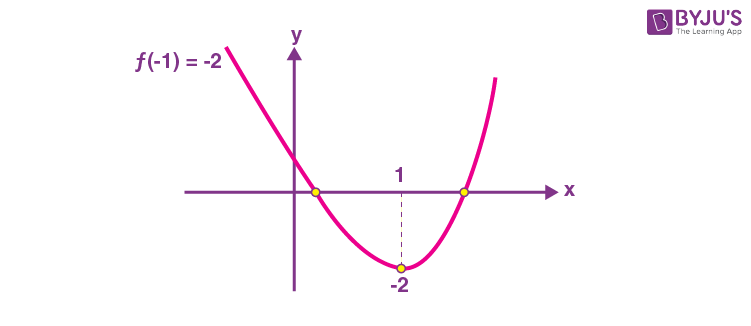⇒ Two solution

7. The lengths of the sides of a triangle are 10 + x2, 10 + x2 and 20 – 2x2. If for x = k, the area of the triangle is maximum, then 3k2 is equal to :

(A) 5

(B) 8

(C) 10

(D) 12

Sol.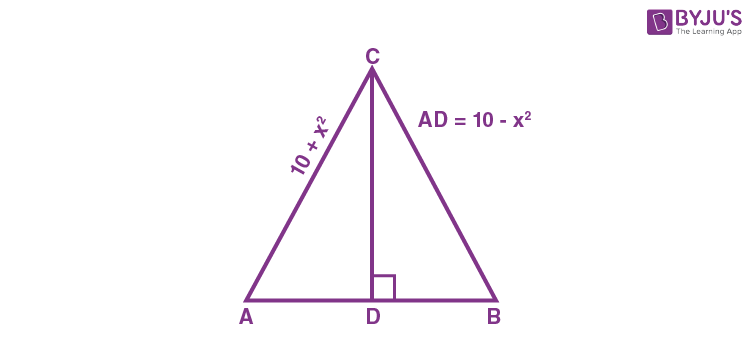$$\begin{array}{l}CD=\sqrt{\left ( 10+x^2 \right )^2-\left ( 10-x^2 \right )^2}=2\sqrt{10}\left|x \right|\end{array}$$

Area

$$\begin{array}{l}=\frac{1}{2}\times CD\times AB=\frac{1}{2}\times 2\sqrt{10}\left|x \right|\left ( 20-2x^2 \right )\end{array}$$

$$\begin{array}{l}A=\sqrt{10}\left|x \right|\left ( 10-x^2 \right )\end{array}$$

$$\begin{array}{l}\frac{dA}{dx}=\sqrt{10}\frac{\left|x \right|}{x}\left ( 10-x^2 \right )+\sqrt{10}\left|x \right|\left ( -2x \right )=0\end{array}$$

⇒ 10 – x2 = 2x2

3x2 = 10

x = k

3k2 = 10

8. If

$$\begin{array}{l}\cos^{-1}\left ( \frac{y}{2} \right )=\textup{log}_e\left ( \frac{x}{5} \right )^5,\left|y \right|<2\end{array}$$
then :

(A) x2y′′ + xy′ – 25y = 0

(B) x2y′′ – xy′ – 25y = 0

(C) x2y′′ – xy′+ 25y = 0

(D) x2y′′ + xy′+ 25y = 0

Sol.

$$\begin{array}{l}\cos^{-1}\left ( \frac{y}{2} \right )=\textup{log}_e\left ( \frac{x}{5} \right )^5,\left|y \right|<2\end{array}$$

Differentatingon both side

$$\begin{array}{l}-\frac{1}{\sqrt{1-\left (\frac{y}{2} \right )^2}}\times\frac{y^{‘}}{2}=\frac{5}{\frac{x}{5}}\times\frac{1}{5}\end{array}$$

$$\begin{array}{l}\frac{-xy^{‘}}{2}=5\sqrt{1-\left ( \frac{y}{2} \right )^2}\end{array}$$

Square on both side

$$\begin{array}{l}\frac{x^2y^{‘2}}{4}=25\left ( \frac{4-y^2}{4} \right )\end{array}$$

Diff on both side

$$\begin{array}{l}2xy^{‘2}+2y^{‘}y^{”}x^2=-25\times2yy^{‘}\end{array}$$

xy′ + y′′x2 + 25y = 0

9. If

$$\begin{array}{l}\int\frac{\left ( x^2+1 \right )e^x}{\left ( x+1 \right )^2}dx=f\left ( x \right )e^x+C\end{array}$$
where C is a constant, then
$$\begin{array}{l}\frac{d^3f}{dx^3}\end{array}$$
at x = 1 is equal to :

$$\begin{array}{l}(A)\ -\frac{3}{4} \end{array}$$

$$\begin{array}{l}(B)\ \frac{3}{4} \end{array}$$

$$\begin{array}{l}(C)\ -\frac{3}{2} \end{array}$$

$$\begin{array}{l}(D)\ \frac{3}{2} \end{array}$$

Sol.

$$\begin{array}{l}I=\int\frac{e^x\left ( x^2+1 \right )}{\left ( x+1 \right )^2}dx=f\left ( x \right )e^x+c\end{array}$$

$$\begin{array}{l}I=\int\frac{e^x\left ( x^2-1+1+1 \right )}{\left ( x+1 \right)^2}dx\end{array}$$

$$\begin{array}{l}=\int e^x\left [ \frac{x-1}{x+1}+\frac{2}{\left ( x+1 \right )^2} \right ]dx\end{array}$$

$$\begin{array}{l}=e^x\left ( \frac{x-1}{x+1} \right )+c\end{array}$$

$$\begin{array}{l}\therefore f\left ( x \right ) =\frac{x-1}{x+1}\end{array}$$

$$\begin{array}{l}f\left ( x \right ) =1-\frac{2}{x+1}\end{array}$$

$$\begin{array}{l}f’\left ( x \right ) =2\left ( \frac{1}{x+1} \right )^2\end{array}$$

$$\begin{array}{l}f”\left ( x \right ) =-4\left ( \frac{1}{x+1} \right )^3\end{array}$$

$$\begin{array}{l}f”’\left ( x \right ) =\frac{12}{\left ( x+1 \right )^4}\end{array}$$

for x = 1

$$\begin{array}{l}f”’\left ( 1 \right ) =\frac{12}{2^4}=\frac{12}{16}=\frac{3}{4}\end{array}$$

10. The value of the integral

$$\begin{array}{l}\displaystyle\int\limits_{-2}^2\frac{\left|x^3+x \right|}{\left (e^{x\left|x\right|}+1 \right ) }dx\end{array}$$
is equal to:

(A) 5e2

(B) 3e–2

(C) 4

(D) 6

Sol.

$$\begin{array}{l}I=\displaystyle\int\limits_{-2}^2\frac{\left|x^3+x \right|}{e^{x\left|x\right|}+1 }dx….(i)\end{array}$$

$$\begin{array}{l}I=\displaystyle\int\limits_{-2}^2\frac{\left|x^3+x \right|}{e^{-x\left|x\right|}+1 }dx….(ii)\end{array}$$

$$\begin{array}{l}2I=\displaystyle\int\limits_{-2}^2\left|x^3+x \right|dx\end{array}$$

$$\begin{array}{l}2I=2\displaystyle\int\limits_0^2\left ( x^3+x \right )dx\end{array}$$

$$\begin{array}{l}I=\displaystyle\int\limits_0^2\left ( x^3+x \right )dx\end{array}$$

$$\begin{array}{l}=\left.\begin{matrix}\frac{x^4}{4}+\frac{x^2}{2}\end{matrix}\right\]_0^2\end{array}$$

$$\begin{array}{l}=\left ( \frac{16}{4}+\frac{4}{2} \right )-0\end{array}$$

= 4 + 2 = 6

11. If

$$\begin{array}{l}\frac{dy}{dx}+\frac{2^{x-y}\left ( 2^y-1 \right )}{2^x-1}=0,x,y>0,y\left ( 1 \right ) =1\end{array}$$
, then y(2) is equal to :

(A) 2 + log2 3

(B) 2 + log3 2

(C) 2 – log3 2

(D) 2 – log2 3

Sol.

$$\begin{array}{l}\frac{dy}{dx}+\frac{2^{x-y}\left ( 2^y-1 \right )}{2^x-1}=0\end{array}$$
, x, y> 0, y(1) = 1

$$\begin{array}{l}\frac{dy}{dx}=-\frac{2^{x}\left ( 2^y-1 \right )}{2^y\left ( 2^x-1 \right )}\end{array}$$

$$\begin{array}{l}\int\frac{2^y}{2^y-1}dy=-\int\frac{2^x}{2^x-1}dx\end{array}$$

$$\begin{array}{l}=\frac{\textup{log}_e\left ( 2^y-1 \right )}{\textup{log}_e2}=-\frac{\textup{log}_e\left (2^x-1 \right )}{\textup{log}_e2}+\frac{\textup{log}_ec}{\textup{log}_e2}\end{array}$$

= |(2y – 1)(2x – 1)| = c

y(1) = 1

c = 1

= |(2y – 1)(2x – 1)| = 1

For x = 2

|(2y – 1)3| = 1

$$\begin{array}{l}2^y-1=\frac{1}{3}\Rightarrow 2y=\frac{4}{3} \end{array}$$

Taking log to base 2.

∴ y = 2 – log2 3

12. In an isosceles triangle ABC, the vertex A is (6, 1) and the equation of the base BC is 2x + y = 4. Let the point B lie on the line x + 3y = 7. If (α, β) is the centroid of ΔABC, then 15(α + β) is equal to :

(A) 39

(B) 41

(C) 51

(D) 63

Sol.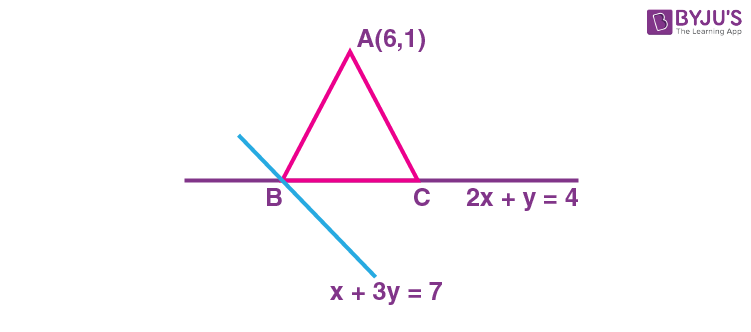$$\begin{array}{l}\left.\begin{matrix}2x+y=4 \\2x+6y=14\end{matrix}\right\}y=2, x=3\end{array}$$

B(1, 2)

Let C(k, 4 – 2k)

Now AB2 = AC2

52 + (–1)2 = (6 – k)2 + (–3 + 2k)2

⇒ 5k2 – 24k + 19 = 0

(5k – 19)(k – 1) = 0

$$\begin{array}{l}\Rightarrow k=\frac{19}{5}\end{array}$$

$$\begin{array}{l}C\left ( \frac{19}{5},-\frac{18}{5} \right )\end{array}$$

Centroid (α, β)

$$\begin{array}{l}\alpha=\frac{6+1+\frac{19}{5}}{3}=\frac{18}{5}\end{array}$$

$$\begin{array}{l}\beta=\frac{1+2-\frac{18}{5}}{3}=-\frac{1}{5}\end{array}$$

Now 15(α + β)

$$\begin{array}{l}15\left ( \frac{17}{5} \right )=51\end{array}$$

13. Let the eccentricity of an ellipse

$$\begin{array}{l}\frac{x^2}{a^2}+\frac{y^2}{b^2}=1,a>b, \end{array}$$
be
$$\begin{array}{l}\frac{1}{4} \end{array}$$
. If this ellipse passes through the point
$$\begin{array}{l}\left ( -4\sqrt{\frac{2}{5}},3 \right )\end{array}$$
, then a2 + b2 is equal to :

(A) 29

(B) 31

(C) 32

(D) 34

Sol.

$$\begin{array}{l}\frac{x^2}{a^2}+\frac{y^2}{b^2}=1\end{array}$$

$$\begin{array}{l}\Rightarrow \frac{\left ( -4\sqrt{\frac{2}{5}} \right )^2}{a^2}+\frac{32}{b^2}=1 \end{array}$$

$$\begin{array}{l}\frac{32}{5a^2}+\frac{9}{b^2}=1 \end{array}$$
…(i)

a2(1 – e2) = b2

$$\begin{array}{l}a^2\left ( 1-\frac{1}{16} \right )=b^2\end{array}$$

15a2 = 16b2

$$\begin{array}{l}a^2=\frac{16b^2}{15} \end{array}$$

From (i)

$$\begin{array}{l}\frac{6}{b^2}+\frac{9}{b^2}=1 \Rightarrow b^2=15\end{array}$$
&a2 = 16

a2 + b2 = 15 + 16 = 31

14. If two straight lines whose direction cosines are given by the relations l + m – n = 0, 3l2 + m2 + cnl = 0 are parallel, then the positive value of cis :

(A) 6

(B) 4

(C) 3

(D) 2

Sol. l + mn = 0 ⇒n = l + m

3l2 + m2 + cnl = 0

3l2 + m2 + cl(l + m) = 0

= (3 + c)l2 + clm + m2 = 0

$$\begin{array}{l}=\left ( 3+c \right ) \left ( \frac{I}{m} \right )^2+c\left ( \frac{I}{m} \right )+1=0\end{array}$$

∵ Lines are parallel

D = 0

c2 – 4(3 + c) = 0

c2 – 4c – 12 = 0

(c – 4)(c + 3) = 0

c = 4 (as c> 0)

15. Let

$$\begin{array}{l}\vec{a}=\hat{i}+\hat{j}-\hat{k} \end{array}$$
and
$$\begin{array}{l}\vec{c}=2\hat{i}-3\hat{j}+2\hat{k} \end{array}$$
. Then the number of vectors
$$\begin{array}{l}\vec{b}\end{array}$$
such that
$$\begin{array}{l}\vec{b}\times\vec{c}=\vec{a}\end{array}$$
and
$$\begin{array}{l}\left|\vec{b} \right|\in\left\{1,2,\dots,10 \right\}\end{array}$$
is :

(A) 0

(B) 1

(C) 2

(D) 3

Sol.

$$\begin{array}{l}\vec{a}=\hat{i}+\hat{j}-\hat{k}\end{array}$$

$$\begin{array}{l}\vec{c}=2\hat{i}-3\hat{j}+2\hat{k}\end{array}$$

Now,

$$\begin{array}{l}\vec{b}\times\vec{c}=\vec{a}\end{array}$$

$$\begin{array}{l}\vec{c}\cdot\left ( \vec{b}\times\vec{c} \right )=\vec{c}\cdot\vec{a}\end{array}$$

$$\begin{array}{l}\vec{c}\cdot\vec{a}=0\end{array}$$

$$\begin{array}{l}\Rightarrow \left ( \hat{i}+\hat{j}-\hat{k} \right )\left ( 2\hat{i}-3\hat{j}+2\hat{k} \right )=0\end{array}$$

= 2 – 3 – 2 = 0

⇒ –3 = 0 (Not possible)

⇒ No possible value of

$$\begin{array}{l}\vec{b} \end{array}$$
is possible.

16. Five numbers, x1, x2, x3, x4, x5 are randomly selected from the numbers 1, 2, 3,….., 18 and are arranged in the increasing order (x1<x2<x3<x4<x5). The probability that x2 = 7 and x4 = 11 is:

(A)

$$\begin{array}{l}\frac{1}{136} \end{array}$$
(B)
$$\begin{array}{l}\frac{1}{72} \end{array}$$

(C)

$$\begin{array}{l}\frac{1}{68} \end{array}$$
(D)
$$\begin{array}{l}\frac{1}{34} \end{array}$$

Sol. Total cases=18C5

Favourable cases

$$\begin{array}{l}\begin{matrix}^6C_1 & ^3C_1 &^7C_1\\\left ( \textup{Select}~x_1 \right ) & \left ( \textup{Select}~x_3 \right )&\left ( \textup{Select}~x_5 \right )\\\end{matrix}\end{array}$$

$$\begin{array}{l}P=\frac{6\cdot3\cdot7}{^{18}C_5}=\frac{1}{68}\end{array}$$

17. Let X be a random variable having binomial distribution B(7, p). If P(X = 3) = 5P(X = 4), then the sum of the mean and the variance of X is:

(A)

$$\begin{array}{l}\frac{105}{16}\end{array}$$

(B)

$$\begin{array}{l}\frac{7}{16}\end{array}$$

(C)

$$\begin{array}{l}\frac{77}{36}\end{array}$$

(D)

$$\begin{array}{l}\frac{49}{16}\end{array}$$

Sol. Given P(X = 3) = 5P(X = 4) and n = 7

$$\begin{array}{l}^7C_3p^3q^4=5\cdot^7C_4p^4q^3\end{array}$$

q = 5p and also p + q = 1

$$\begin{array}{l}p=\frac{1}{6}~\textup{and}~q=\frac{5}{6}\end{array}$$

Mean

$$\begin{array}{l}=\frac{7}{6} \end{array}$$
and variance
$$\begin{array}{l}=\frac{35}{36} \end{array}$$

Mean + Variance

$$\begin{array}{l}=\frac{7}{6}+\frac{35}{36}=\frac{77}{36} \end{array}$$

18. The value of

$$\begin{array}{l}\cos\left ( \frac{2\pi}{7} \right )+\cos\left ( \frac{4\pi}{7} \right )+\cos\left ( \frac{6\pi}{7} \right )\end{array}$$
is equal to:

(A) –1

(B)

$$\begin{array}{l}-\frac{1}{2} \end{array}$$

(C)

$$\begin{array}{l}-\frac{1}{3} \end{array}$$

(D)

$$\begin{array}{l}-\frac{1}{4} \end{array}$$

Sol.

$$\begin{array}{l}\cos\frac{2\pi}{7}+\cos\frac{4\pi}{7}+\cos\frac{6\pi}{7}=\frac{\sin3\left ( \frac{\pi}{7} \right )}{\sin\frac{\pi}{7}}\cos\frac{\left ( \frac{2\pi}{7}+\frac{6\pi}{7} \right )}{2}\end{array}$$

$$\begin{array}{l}=\frac{\sin\left ( \frac{3\pi}{7}\right)\cdot\cos\left ( \frac{4\pi}{7} \right )}{\sin\left ( \frac{\pi}{7} \right )}\end{array}$$

$$\begin{array}{l}=\frac{2\sin\frac{4\pi}{7}\cos\frac{4\pi}{7}}{2\sin\frac{\pi}{7}} \end{array}$$

$$\begin{array}{l}=\frac{\sin\left ( \frac{8\pi}{7} \right )}{2\sin\frac{\pi}{7}}=\frac{-\sin\frac{\pi}{7}}{2\sin\frac{\pi}{7}}=\frac{-1}{2}\end{array}$$

19.

$$\begin{array}{l}\sin^{-1}\left ( \sin\frac{2\pi}{3} \right )+\cos^{-1}\left ( \cos\frac{7\pi}{6} \right )+\tan^{-1}\left ( \tan\frac{3\pi}{4} \right )\end{array}$$
is equal to:

(A)

$$\begin{array}{l}\frac{11\pi}{12}\end{array}$$

(B)

$$\begin{array}{l}\frac{17\pi}{12}\end{array}$$

(C)

$$\begin{array}{l}\frac{31\pi}{12}\end{array}$$

(D)

$$\begin{array}{l}-\frac{3\pi}{4}\end{array}$$

Sol.

$$\begin{array}{l}\sin^{-1}\left ( \frac{\sqrt{3}}{2} \right )+\cos^{-1}\left ( \frac{-\sqrt{3}}{2} \right )+\tan^{-1}\left ( -1 \right )\end{array}$$

$$\begin{array}{l}=\frac{\pi}{3}+\frac{5\pi}{6}-\frac{\pi}{4}\end{array}$$

$$\begin{array}{l}=\frac{4\pi+10\pi-3\pi}{12}=\frac{11\pi}{12}\end{array}$$

20. The boolean expression (~(p q)) ∨q is equivalent to:

(A) q→ (p q)

(B) pq

(C) p→ (pq)

(D) p→ (pq)

Sol. Making truth table

 p q p ∧ q ~p ∧ q (~(p ∧ q)) ∨ q p ∨ q p → q p → (p ∨ q) T T T F T T T T T F F T T T F T F T F T T T T T F F F T T F T T Tautology Tautology

$$\begin{array}{l}\therefore \left ( \sim \left ( p\wedge q \right ) \right )\vee q\equiv p\rightarrow\left ( p\vee q \right )\end{array}$$

SECTION – B

Numerical Value Type Questions: This section contains 10 questions. In Section B, attempt any five questions out of 10. The answer to each question is a NUMERICAL VALUE. For each question, enter the correct numerical value (in decimal notation, truncated/rounded-off to the second decimal place; e.g. 06.25, 07.00, –00.33, –00.30, 30.27, –27.30) using the mouse and the on-screen virtual numeric keypad in the place designated to enter the answer.

1. Let ƒ :RR be a function defined by

$$\begin{array}{l}f\left ( x \right )=\frac{2e^{2x}}{e^{2x}+e^x} \end{array}$$
Then
$$\begin{array}{l}f\left ( \frac{1}{100} \right )+f\left ( \frac{2}{100} \right )+f\left ( \frac{3}{100} \right )+\dots+f\left ( \frac{99}{100} \right )\end{array}$$
is equal to ________.

Sol.

$$\begin{array}{l}f\left ( x \right )=\frac{2e^{2x}}{e^{2x}+e^x}~\textup{and}~f\left ( 1-x \right )=\frac{2e^{2-2x}}{e^{2-2x}+e^{1-x}}\end{array}$$

$$\begin{array}{l}\frac{f\left ( x \right )+f\left ( 1-x \right )}{2}=1\end{array}$$

i.e. f(x) + f(1 – x) = 2

$$\begin{array}{l}f\left ( \frac{1}{100} \right ) +f\left ( \frac{2}{100} \right )+\dots+f\left ( \frac{99}{100} \right )\end{array}$$

$$\begin{array}{l}=\displaystyle\sum\limits_{x=1}^{49}f\left ( \frac{x}{100} \right )+f\left ( 1-\frac{x}{100} \right )+f\left ( \frac{1}{2} \right )\end{array}$$

= 49 × 2 + 1 = 99

2. If the sum of all the roots of the equation

$$\begin{array}{l}e^{2x} – 11e^x – 45e^{x} +\frac{81}{2}=0\end{array}$$
is logep, then p is equal to _____.

Sol. Let ex = t then equation reduces to

$$\begin{array}{l}t^2-11t-\frac{45}{t}+\frac{81}{2}=0 \end{array}$$

⇒ 2t3 – 22t2 + 81t – 45 = 0 …(i)

if roots of

$$\begin{array}{l}e^{2x}-11e^x-45e^{-x}+\frac{81}{2}=0 \end{array}$$
are α, β, γ then roots of (i) will be
$$\begin{array}{l}e^{\alpha_1}e^{\alpha_2}e^{\alpha_3}\end{array}$$
using product of roots

$$\begin{array}{l}e^{\alpha_1+\alpha_2+\alpha_3}=45\end{array}$$

⇒ α1 + α2 + α3 = ln 45 ⇒ p = 45

3. The positive value of the determinant of the matrix A, whose

$$\begin{array}{l}Adj\left ( Adj\left ( A \right ) \right )=\begin{pmatrix}14 & 28 & -14 \\-14 & 14 & 28 \\28 & -14 & 14 \\\end{pmatrix}\end{array}$$
, is __________.

Sol.

$$\begin{array}{l}\left|adj\left ( adj\left ( A \right ) \right ) \right|=\left|A \right|^{2^2}=\left|A \right|^4\end{array}$$

$$\begin{array}{l}\left|A \right|^4=\begin{vmatrix}14 & 28 & -14 \\-14 & 14 & 28 \\28 & -14 & 14 \\\end{vmatrix}\end{array}$$

$$\begin{array}{l}=\left ( 14 \right )^3\begin{vmatrix}1 & 2 & -1 \\-1 & 1 & 2 \\2 & -1 & 1 \\\end{vmatrix}\end{array}$$

$$\begin{array}{l}=\left ( 14 \right )^3\left ( 3-2\left ( -5 \right )-1\left ( -1 \right ) \right )\end{array}$$

$$\begin{array}{l}\left|A \right|^4=\left ( 14 \right )^4\Rightarrow \left| A\right|=14\end{array}$$

4. The number of ways, 16 identical cubes, of which 11 are blue and rest are red, can be placed in a row so that between any two red cubes there should be at least 2 blue cubes, is _________.

Sol. First we arrange 5 red cubes in a row and assume x1, x2, x3, x4, x5 and x6 number of blue cubes between them

Here, x1 + x2 + x3 + x4 + x5 + x6 = 11

and x2, x3, x4, x5≥ 2

So x1 + x2 + x3 + x4 + x5 + x6 = 3

No. of solutions = 8C5 = 56

5. If the coefficient of x10 in the binomial expansion of

$$\begin{array}{l}\left ( \frac{\sqrt{x}}{5^{\frac{1}{4}}}+\frac{\sqrt{5}}{x^{\frac{1}{3}}} \right )^{60}\end{array}$$
is 5k.l, where l, kN and l is co-prime to 5, then k is equal to ___________.

Sol.

$$\begin{array}{l}T_{r+1}=60_{C_r}\left ( x^{\frac{1}{2}} \right )^{60-r}\left ( x^{-\frac{1}{3}} \right )^r\left ( 5^{\frac{-1}{4}} \right )^{60-r}\left ( 5^\frac{1}{2} \right )^r\end{array}$$

for

$$\begin{array}{l}x^{10}\frac{60-r}{2}-\frac{r}{3}=10 \end{array}$$

⇒ 180 – 3r – 2r = 60

r = 24

∴ Coeff. of

$$\begin{array}{l}x^{10}=\frac{^{60}C_{24}}{5^9} 5^{12}=5^k~~I\end{array}$$

as l and 5 are coprime

k = 3 + exponent of 5 in 60C24

$$\begin{array}{l}=3+\left ( \left [\frac{60}{5} \right ]+\left [ \frac{60}{5^2} \right ]-\left [ \frac{24}{5} \right ]-\left [ \frac{24}{5^2} \right ]-\left [ \frac{36}{5} \right ]-\left [ \frac{36}{5^2} \right ] \right. \end{array}$$

= 3 + (12 + 2 – 4 – 0 – 7 – 1)

= 3 + 2 = 5

6. Let

$$\begin{array}{l}A_1=\left\{\left ( x,y \right ):\left|x \right|\leq y^2,\left|x \right|+2y\leq8 \right\} \end{array}$$
and

$$\begin{array}{l}A_2=\left\{\left ( x,y \right ):\left|x \right|+ \left|y \right|\leq k \right\} \end{array}$$
. If 27(Area A1) = 5(Area A2), then k is equal to :

Sol.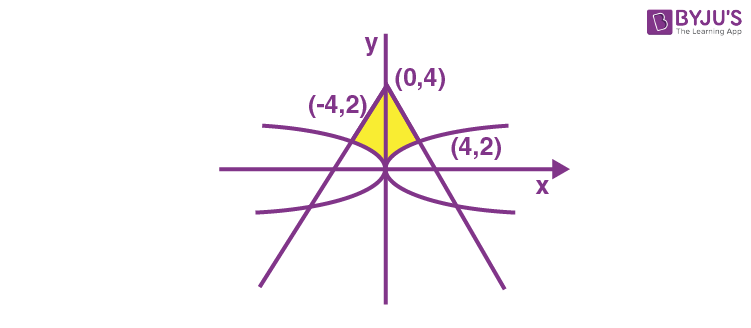Required area (above x-axis)

$$\begin{array}{l}A_1=2\displaystyle\int\limits_0^4\left ( \frac{8-x}{2}-\sqrt{x} \right )dx \end{array}$$

$$\begin{array}{l}=2\left ( 16-\frac{16}{4}-\frac{8}{3/2} \right ) =\frac{40}{3}\end{array}$$

and

$$\begin{array}{l}A_2=4\left ( \frac{1}{2}\cdot k^2 \right )=2k^2\end{array}$$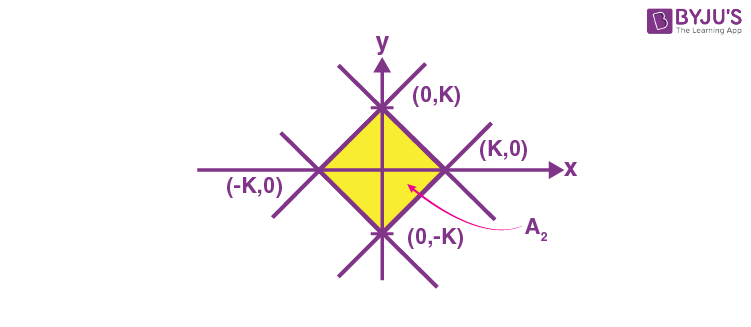$$\begin{array}{l}27\cdot\frac{40}{3}=5\cdot\left ( 2k^2 \right ) \end{array}$$

k = 6

* A1 isWhich tends to infinity if not mentioned above x-axis

7. If the sum of the first ten terms of the series

$$\begin{array}{l}\frac{1}{5}+\frac{2}{65}+\frac{3}{325}+\frac{4}{1025}+\frac{5}{2501}+\dots \end{array}$$
is
$$\begin{array}{l}\frac{m}{n}\end{array}$$
, where m and n are co-prime numbers, then m + n is equal to __________.

Sol.

$$\begin{array}{l}T_r=\frac{r}{\left ( 2r^2 \right )^2+1} \end{array}$$

$$\begin{array}{l}=\frac{r}{\left ( 2r^2+1 \right )^2-\left ( 2r \right )^2} \end{array}$$

$$\begin{array}{l}=\frac{1}{4}\frac{4r}{\left ( 2r^2+2r+1\right )\left ( 2r^2-2r+1 \right )}\end{array}$$

$$\begin{array}{l}S_{10} = \frac{1}{4}\displaystyle\sum\limits_{r=1}^{10}\left ( \frac{1}{\left ( 2r^2-2r+1 \right )}-\frac{1}{\left ( 2r^2+2r+1 \right )} \right )\end{array}$$

$$\begin{array}{l}=\frac{1}{4}\left [1-\frac{1}{5}+\frac{1}{5}-\frac{1}{13}+\dots\dots+\frac{1}{181}-\frac{1}{221} \right ] \end{array}$$

$$\begin{array}{l}S_{10}=\frac{1}{4}\cdot\frac{220}{221}=\frac{55}{221}=\frac{m}{n}\end{array}$$

m + n = 276

8. A rectangle R with end points of one of its sides as (1, 2) and (3, 6) is inscribed in a circle. If the equation of a diameter of the circle is

2xy + 4 = 0, then the area of R is ________.

Sol.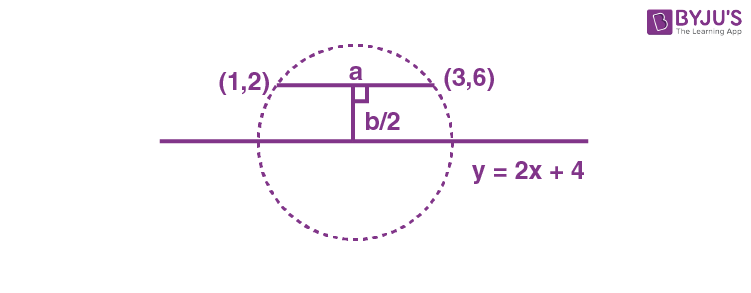As slope of line joining (1, 2) and (3, 6) is 2 given diameter is parallel to side

$$\begin{array}{l}a=\sqrt{\left ( 3-1 \right )^2+\left ( 6-2 \right )^2}=\sqrt{20} \end{array}$$

and

$$\begin{array}{l}\frac{b}{2}=\frac{4}{\sqrt{5}}\Rightarrow b=\frac{8}{\sqrt{5}} \end{array}$$

Area

$$\begin{array}{l}ab=2\sqrt{5}\cdot\frac{8}{\sqrt{5}}=16 \end{array}$$
.

9. A circle of radius 2 unit passes through the vertex and the focus of the parabola y2 = 2x and touches the parabola

$$\begin{array}{l}y=\left ( x-\frac{1}{4} \right )^2+\alpha \end{array}$$
where α> 0. Then (4α – 8)2 is equal to ___________.

Sol.Let the equation of circle be

$$\begin{array}{l}x\left ( x-\frac{1}{2} \right )+y^2+\lambda y=0\end{array}$$

$$\begin{array}{l}x^2+y^2-\frac{1}{2}x+\lambda y=0 \end{array}$$

$$\begin{array}{l}=\sqrt{\frac{1}{16}+\frac{\lambda^2}{4}}=2\end{array}$$

$$\begin{array}{l}\lambda^2=\frac{63}{4}\end{array}$$

$$\begin{array}{l}\left ( x-\frac{1}{4} \right )^2+\left ( y+\frac{\lambda}{2} \right )^2=4 \end{array}$$

∵ This circle and parabola

$$\begin{array}{l}y-\alpha =\left ( x-\frac{1}{4} \right )^2\end{array}$$
touch each other, so

$$\begin{array}{l}\alpha=-\frac{\lambda}{2}+2\end{array}$$

$$\begin{array}{l}\alpha-2=-\frac{\lambda}{2}\end{array}$$

$$\begin{array}{l}\left ( \alpha-2 \right )^2=\frac{\lambda^2}{4}=\frac{63}{16}\end{array}$$

$$\begin{array}{l}\left ( 4\alpha-8 \right )^2=63\end{array}$$

10. Let the mirror image of the point (a, b, c) with respect to the plane 3x – 4y + 12z + 19 = 0 be

(a – 6, β, γ). If a + b + c = 5, then 7β – 9γ is equal to __________.

Sol.

$$\begin{array}{l}\frac{x-a}{3}=\frac{y-b}{-4}=\frac{z-c}{12}=\frac{-2\left ( 3a-4b+12c+19 \right )}{3^2+\left ( -4 \right )^2+12^2} \end{array}$$

$$\begin{array}{l}\frac{x-a}{3}=\frac{y-b}{-4}=\frac{z-c}{12}=\frac{-6a+8b-24c-38}{169} \end{array}$$

$$\begin{array}{l}\left ( x,y,z \right )\equiv\left ( a-6, \beta,\gamma \right )\end{array}$$

$$\begin{array}{l}\frac{\left ( a-b \right )-a}{3}=\frac{\beta-b}{-4}=\frac{\gamma-c}{12}=\frac{-6a+8b-24c-38}{169} \end{array}$$

$$\begin{array}{l}\frac{\beta-b}{-4}=-2\end{array}$$

⇒ β = 8 + b

$$\begin{array}{l}\frac{\gamma-c}{12}=-2 \end{array}$$

⇒ γ = -24 + c

$$\begin{array}{l}\frac{-6a+8b-24c-38}{169}=-2\end{array}$$

⇒ 3a – 4b + 12c = 150….(1)

a + b + c = 5

⇒ 3a + 3b + 3c = 15….(2)

Applying (1) – (2), we get;

-7b + 9c = 135

7b – 9c = -135

7β – 9γ = 7(8 + b) – 9 (–24 + c)

= 56 + 216 + 7b – 9c.

= 56 + 216 – 135 = 137.

### Download PDF of JEE Main 2022 June 27 Shift 1 Maths Paper & Solutions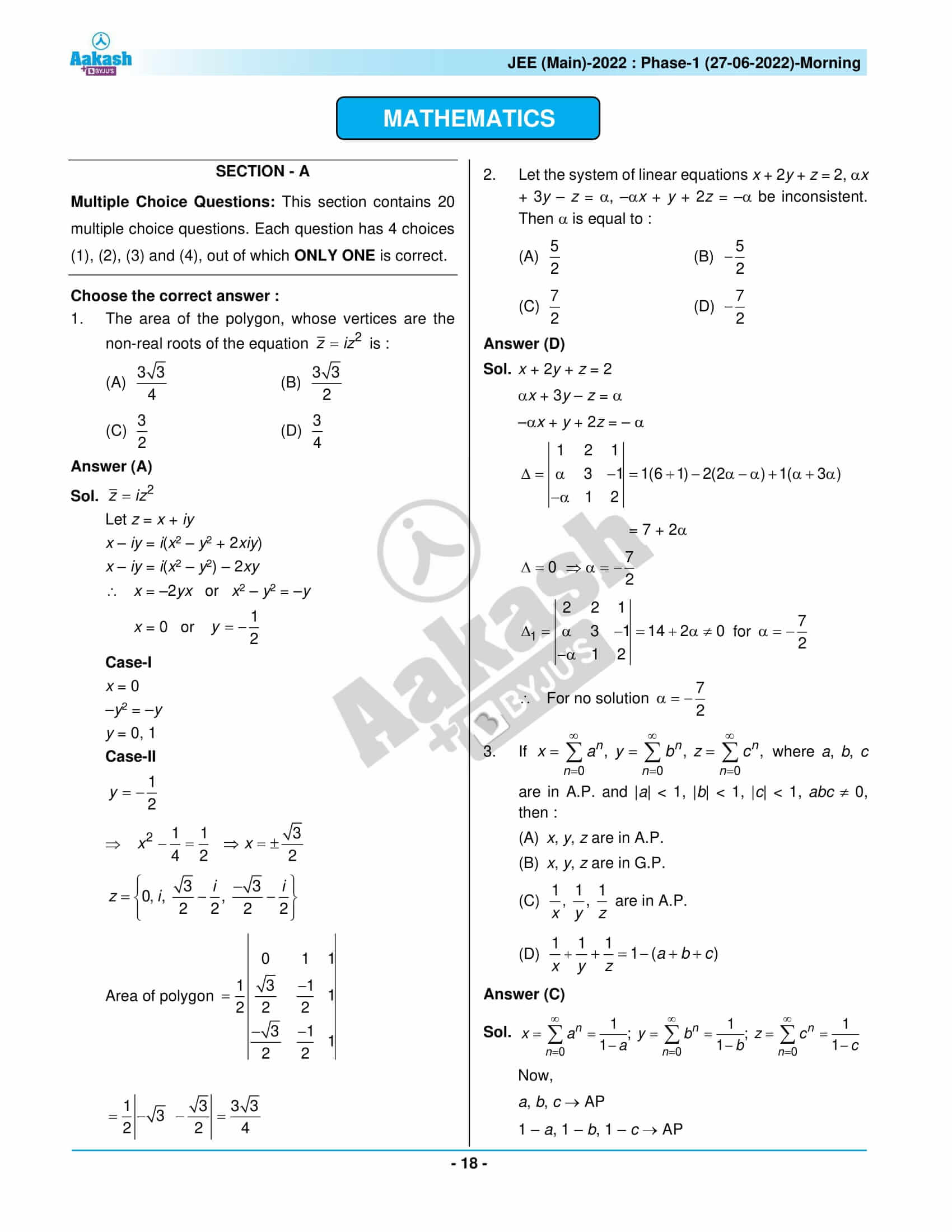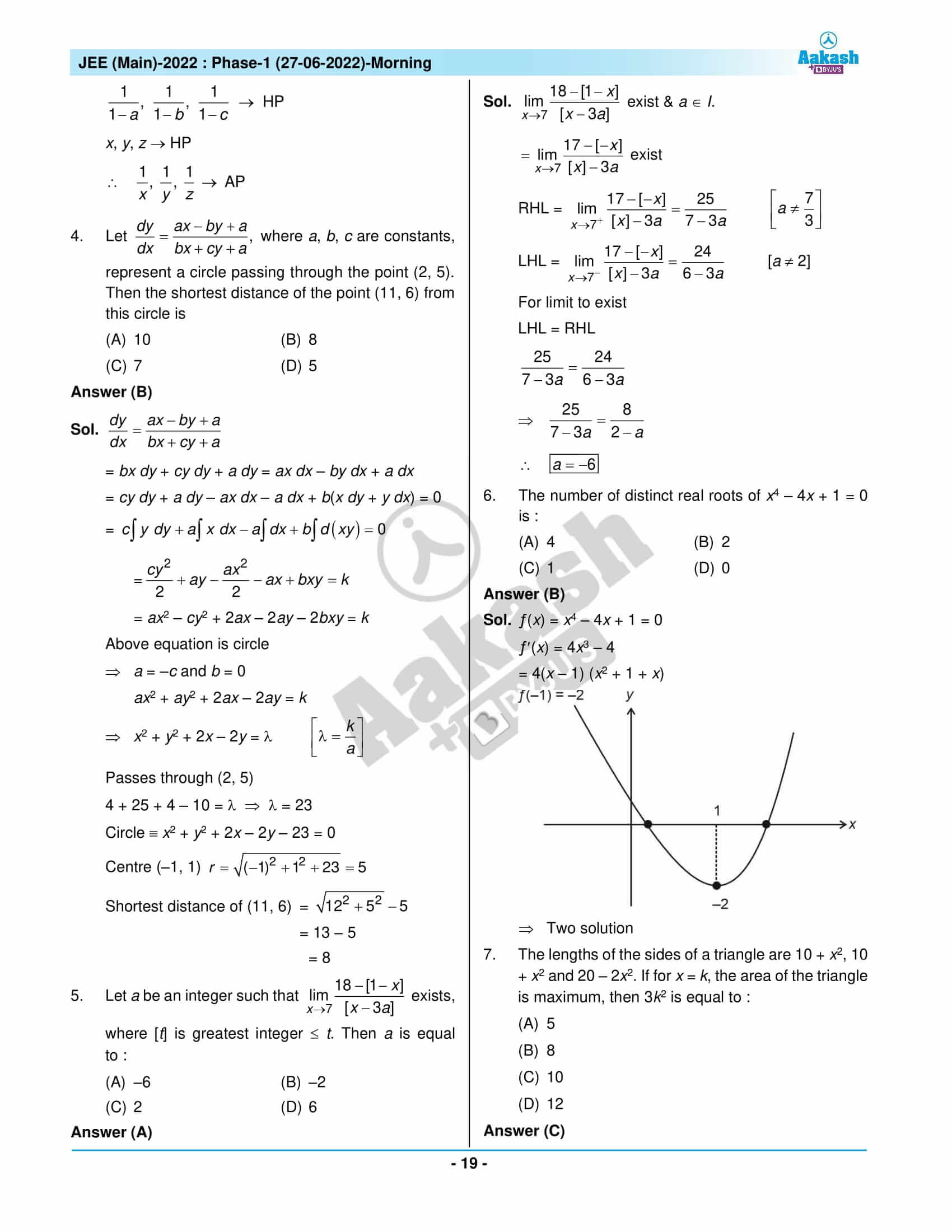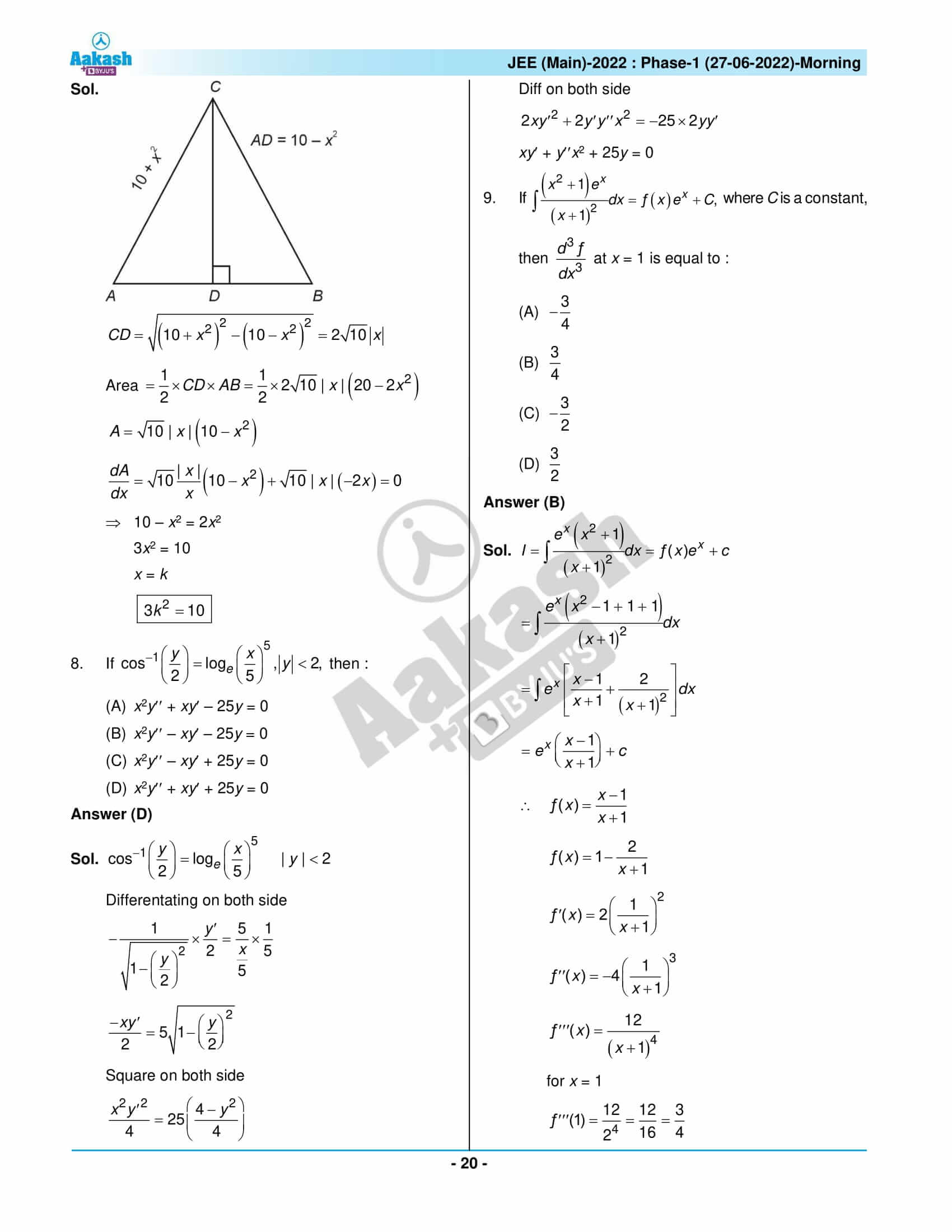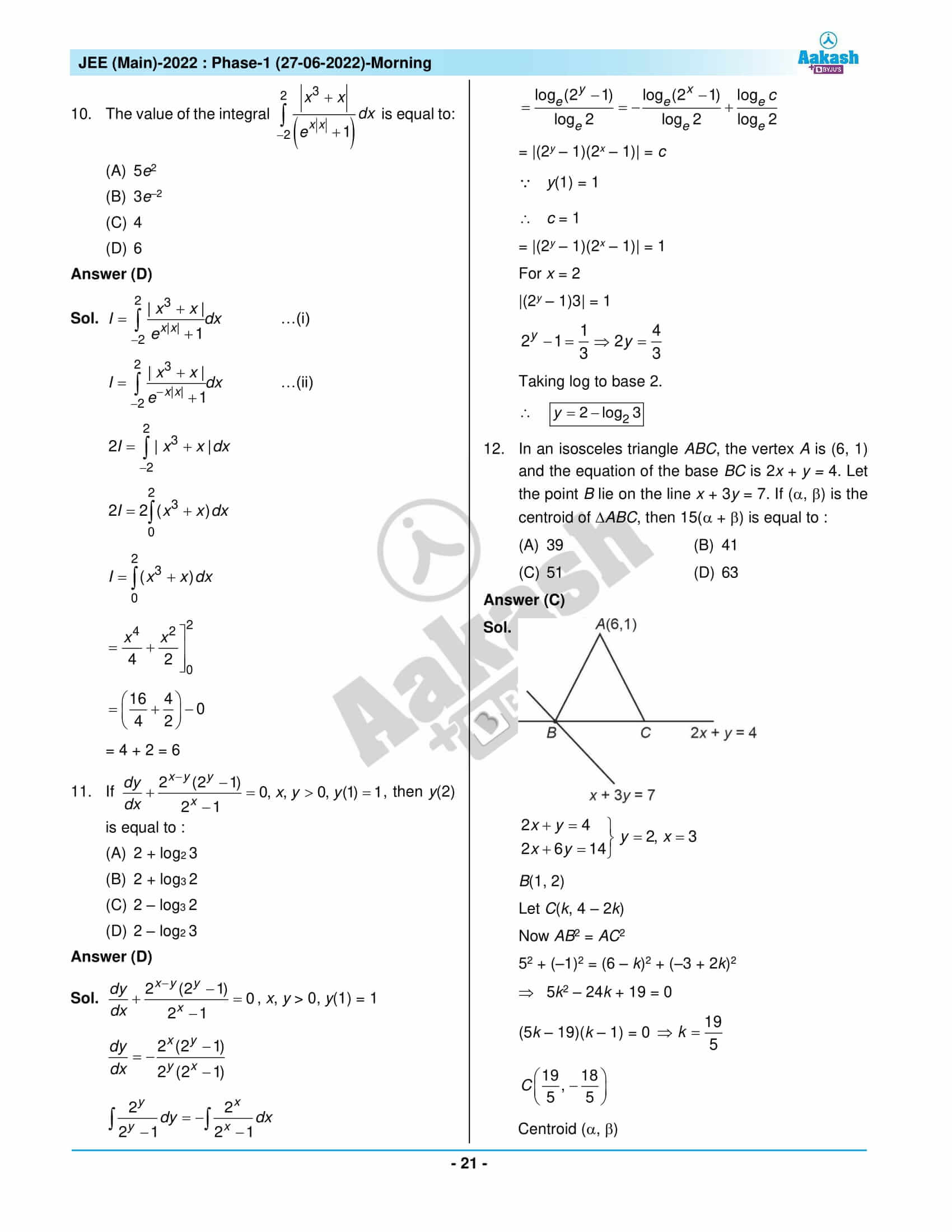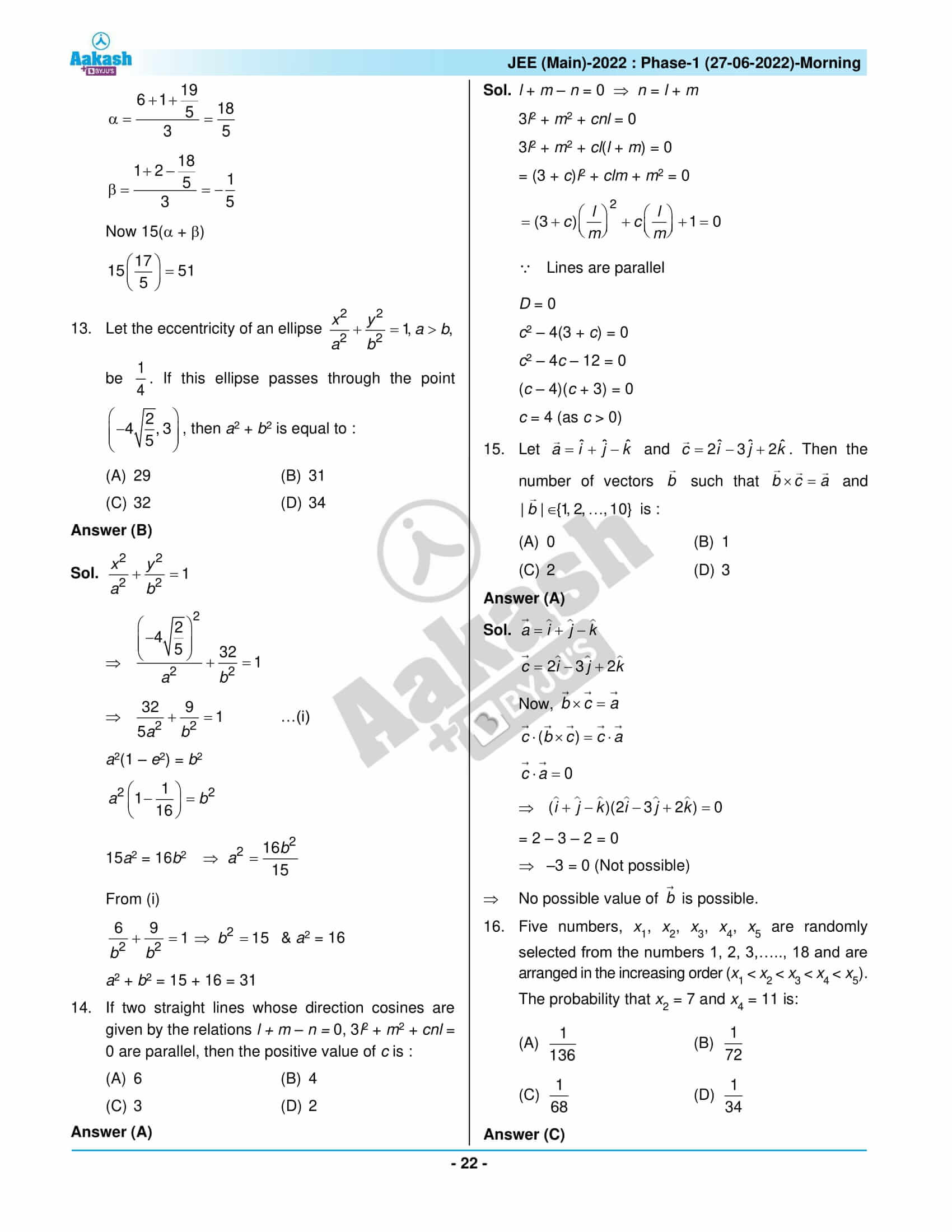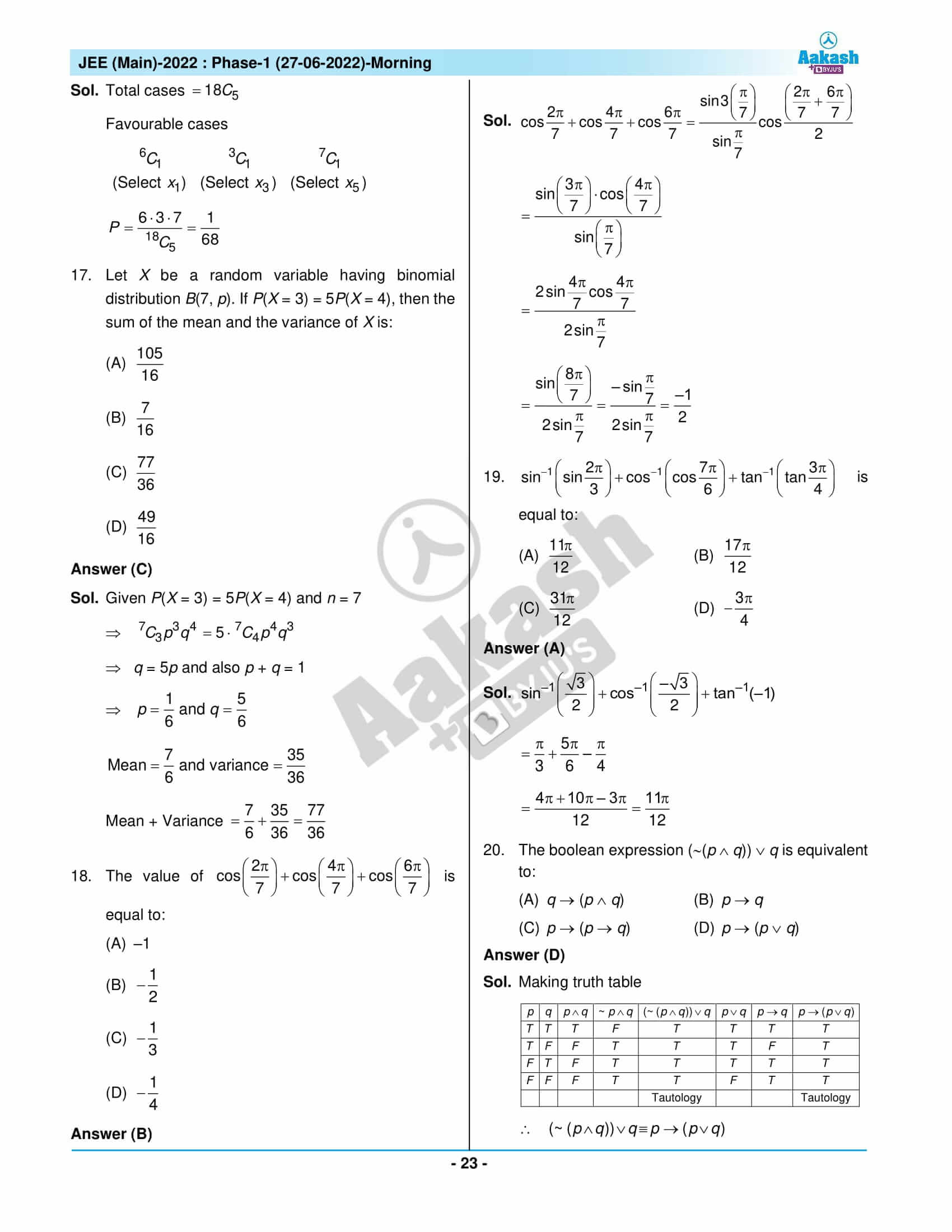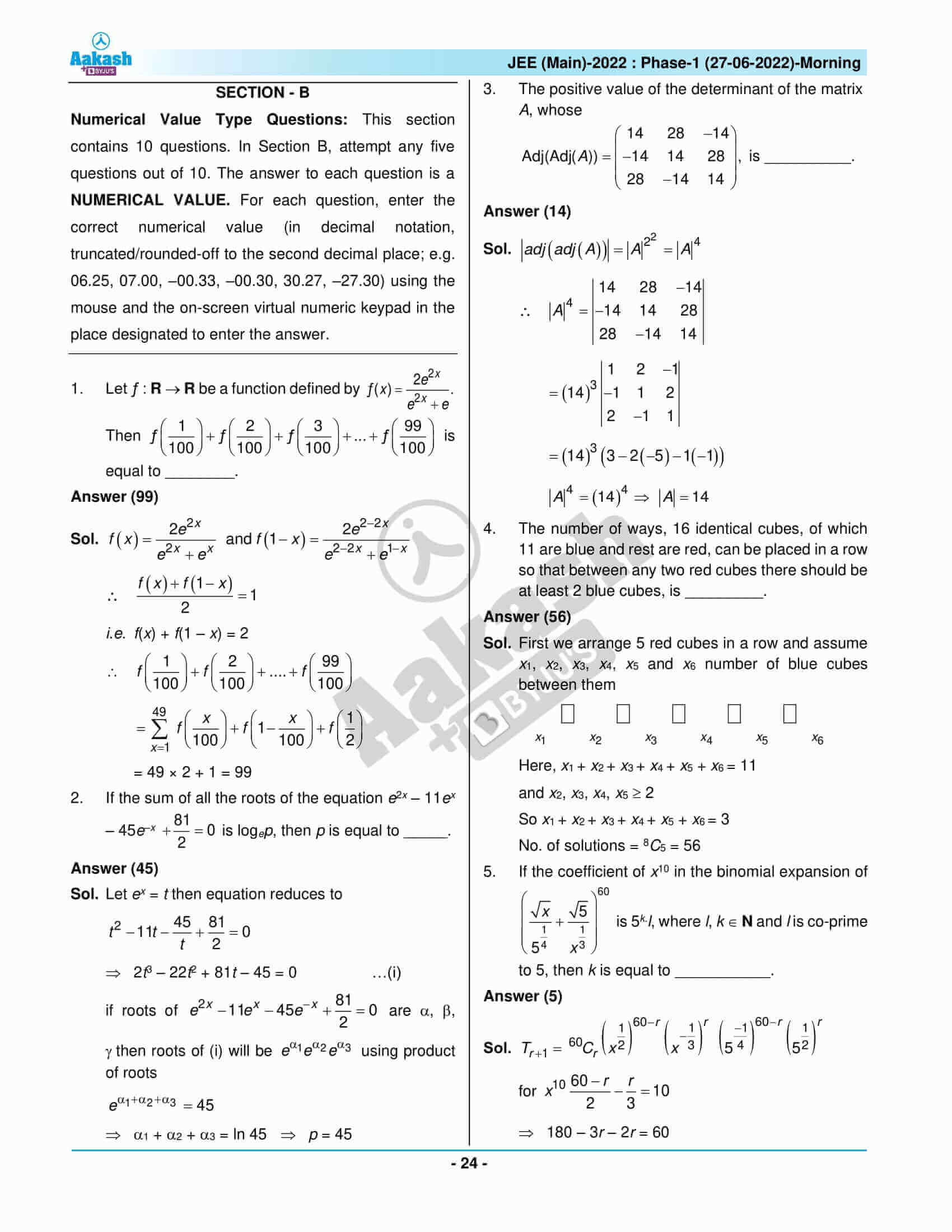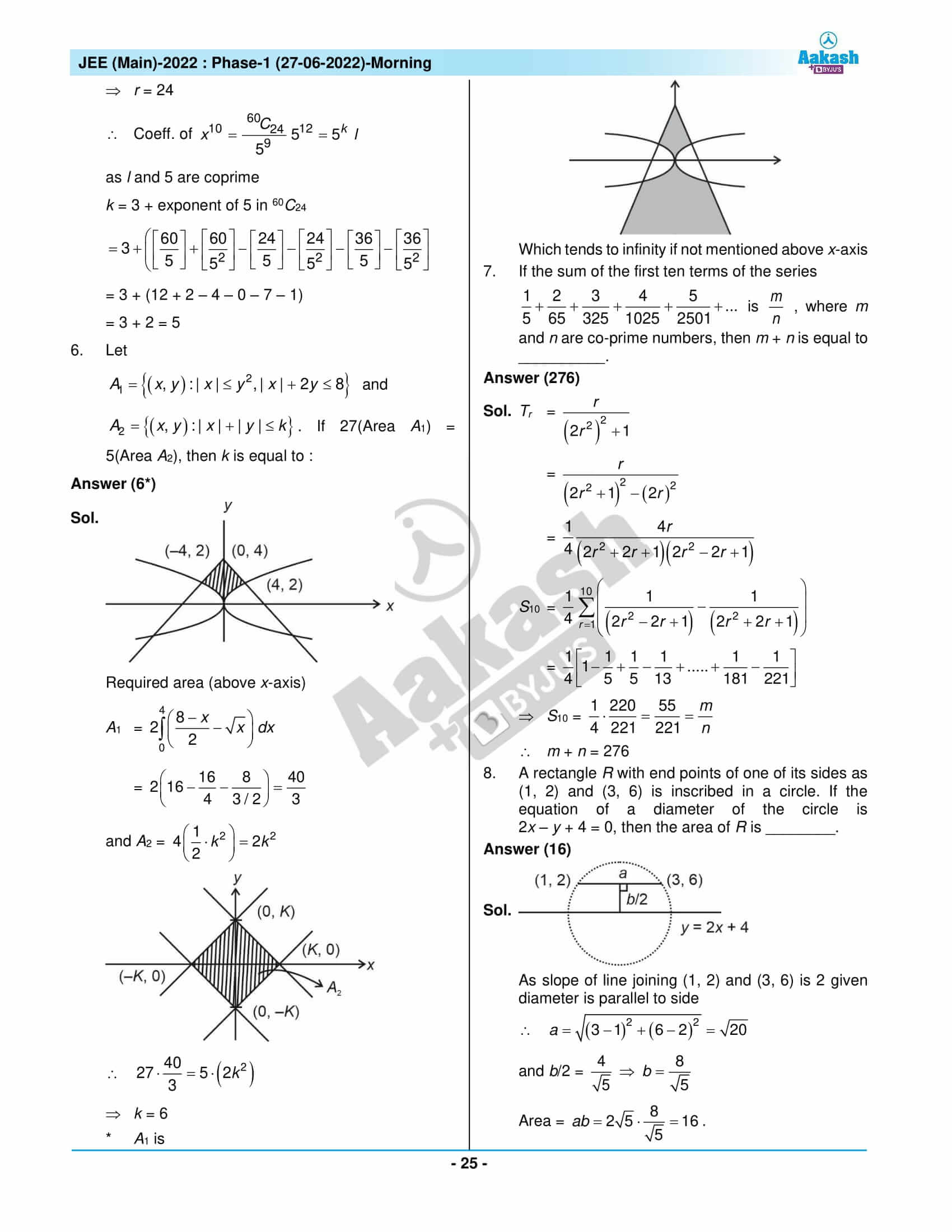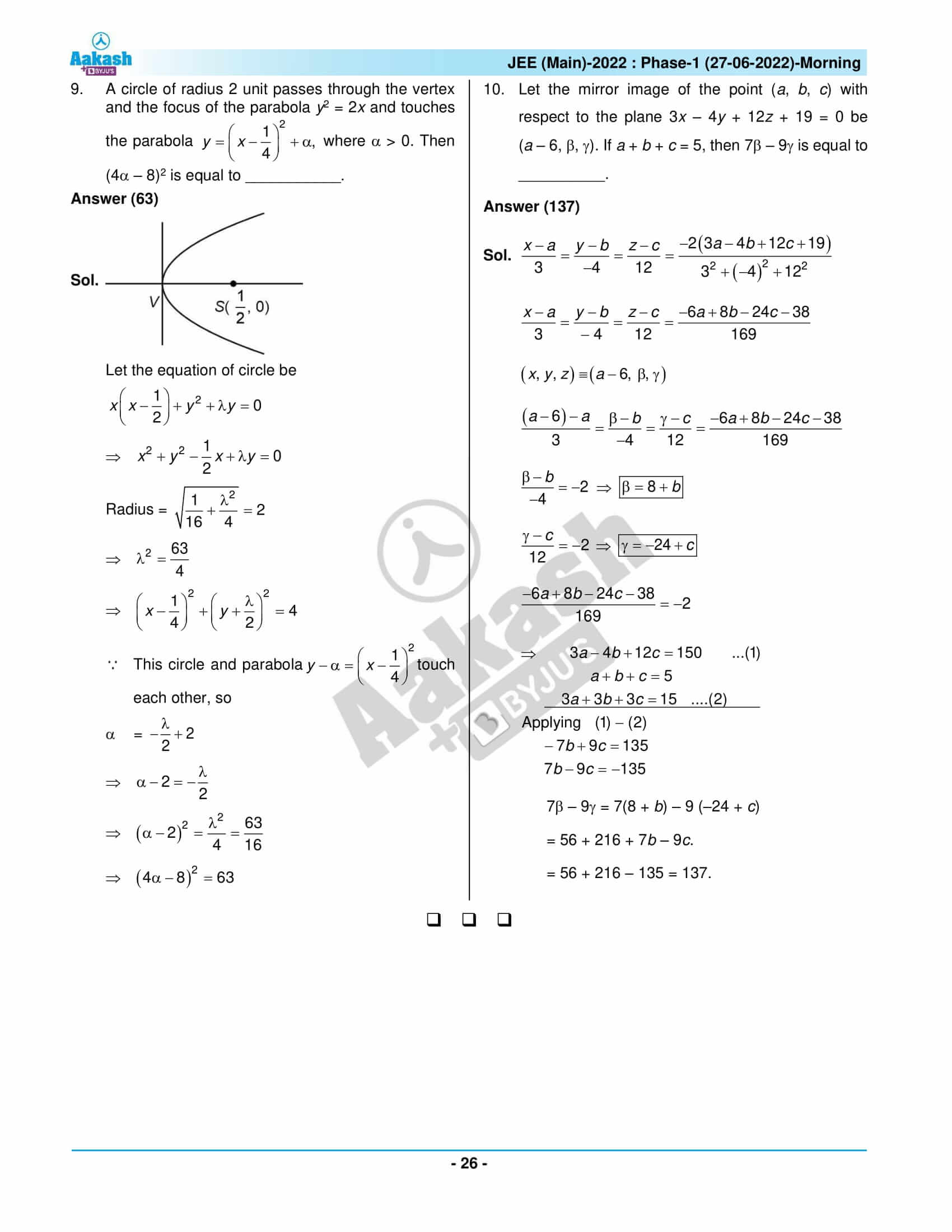## JEE Main 2022 June 27th Shift 1 Question Paper & Solutions

### JEE Main 2022 June 27 Shift 1 Question Paper – Physics Solutions### JEE Main 2022 June 27 Shift 1 Question Paper – Chemistry Solutions### JEE Main 2022 June 27 Shift 1 Question Paper – Maths Solutions## Frequently Asked Questions – FAQs

Q1

### How was the JEE Main 2022 June 27 Shift 1 Maths question paper?

The JEE Main 2022 June 27 Shift 1 Maths question paper was easy to moderate level difficulty. There were 8 easy questions, 11 medium questions and 2 difficult questions in the JEE Main 2022 June 27 Shift 1 Maths question paper.

Q2

### Is there a negative marking in section B of the JEE Main 2022 June 27 Maths Shift 1 question paper?

No. There is no negative marking in section B of the JEE Main 2022 June 27 Maths Shift 1 question paper.

Q3

### How many questions were asked from Class 11 in the JEE Main 2022 Maths June 27 Shift 1 question paper?

There were 10 questions from Class 11 in the JEE Main 2022 Maths June 27 Shift 1 question paper as per the memory-based question paper.

Q4

### What is the overall difficulty level of the JEE Main 2022 Maths June 27 Shift 1 question paper?

The overall difficulty level of JEE Main 2022 June 27 Shift 1 Maths question paper is 1.71 out of 3

Q5

### Were there any questions from Quadratic equations in the JEE Main 2022 June 27 Maths Shift 1 question paper?

Yes. Questions were there from Quadratic equations in the JEE Main 2022 June 27 Maths Shift 1 question paper, and those were easy.